Courses

# Test: Symmetry - 1

## 20 Questions MCQ Test Mathematics (Maths) Class 7 | Test: Symmetry - 1

Description
This mock test of Test: Symmetry - 1 for Class 7 helps you for every Class 7 entrance exam. This contains 20 Multiple Choice Questions for Class 7 Test: Symmetry - 1 (mcq) to study with solutions a complete question bank. The solved questions answers in this Test: Symmetry - 1 quiz give you a good mix of easy questions and tough questions. Class 7 students definitely take this Test: Symmetry - 1 exercise for a better result in the exam. You can find other Test: Symmetry - 1 extra questions, long questions & short questions for Class 7 on EduRev as well by searching above.
QUESTION: 1

### How many lines of symmetries are there in regular pentagon?

Solution:

There are 5 lines in the pentagon and the symmetry line passes from vertex to the middle of the line opposite to the vertex . So, lines of symmetry are 5.

QUESTION: 2

Solution:
QUESTION: 3

### Find the number of lines of symmetry of the following figure: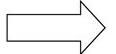Solution:
QUESTION: 4

Find the number of lines of symmetry of the following figure: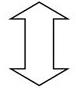Solution:

Yes there are only two lines of symmetry i.e. a horizontal and a vertical line of symmetry, diagonally its not symmetrical.

QUESTION: 5

Find the number of lines of symmetry in regular hexagon.

Solution:
QUESTION: 6

Letter ‘A’ of the English alphabet have reflectional symmetry (i.e., symmetry related to mirror reflection) about.

Solution:

Vertical mirror – A, H, I, M, O, T, U, V, W, X and Y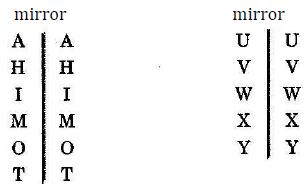QUESTION: 7

Letter ‘B’ of the English alphabet have reflectional symmetry (i.e., symmetry related to mirror reflection) about.

Solution:

Yes , B has a horizontal line of symmetry from the middle , but does not have a vertical line of symmetry, because its not symmetrical with vertical line.

QUESTION: 8

Letter ‘C’ of the English alphabet have reflectional symmetry (i.e., symmetry related to mirror reflection) about.

Solution:

when you’ll place a horizontal mirror you’ll see that C appears C in the mirror.

QUESTION: 9

Letter ‘D’ of the English alphabet have reflectional symmetry (i.e., symmetry related to mirror reflection) about.

Solution:

When you’ll place a horizontal mirror you’ll see that D appears D in the mirror. If you place a vertical mirror D will be laterally inverted.

QUESTION: 10

Letter ‘E’ of the English alphabet have reflectional symmetry (i.e., symmetry related to mirror reflection) about.

Solution:

Horizontal mirror – B, C, D, E, H, I, O and X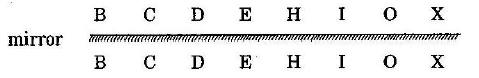QUESTION: 11

Letter ‘G’ of the English alphabet have reflectional symmetry (i.e., symmetry related to mirror reflection) about.

Solution:

Yes , G does not have any line of symmetry , if you draw it horizontally or vertically , its not symmetrical.

QUESTION: 12

Letter ‘H’ of the English alphabet have reflectional symmetry (i.e., symmetry related to mirror reflection) about.

Solution:

Letters of the English alphabet symmetric about both horizontal and vertical mirrors: -

H, I, O and X

The letters when flipped along both the axes (horizontal and vertical) retains the original figure.

QUESTION: 13

Letter ‘I’ of the English alphabet have reflectional symmetry (i.e., symmetry related to mirror reflection) about.

Solution:

‘I’ have both vertical and horizontal symmetry since horizontal division divides ’I’ symmetrical similarly it has a vertical symmetry.

QUESTION: 14

Letter ‘M’ of the English alphabet have reflectional symmetry (i.e., symmetry related to mirror reflection) about.

Solution:
QUESTION: 15

Letter ‘T’ of the English alphabet have reflectional symmetry (i.e., symmetry related to mirror reflection) about.

Solution:

T has only vertical symmetry  since if we draw a horizontal line, we have -on one half but not in other half

QUESTION: 16

Letter ‘T’ of the English alphabet have reflectional symmetry (i.e., symmetry related to mirror reflection) about.

Solution:
QUESTION: 17

Find the number of lines of symmetry in the below figure: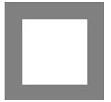Solution:
QUESTION: 18

Find the number of lines of symmetry in the below figure: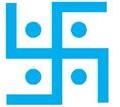Solution:

If you draw a vertical or horizontal or diagonal line you will find that its not symmetrical. Hence, it doesn’t have any lines of symmetry.

QUESTION: 19

Find the number of lines of symmetry in the below figure: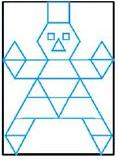Solution:

It has only 1 line of symmetry which is a vertical line of symmetry, as if you draw a horizontal line one half will have a face and one half will have legs which are not symmetrical.

QUESTION: 20

Find the number of lines of symmetry in the below figure: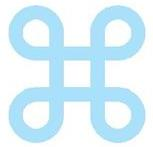Solution: